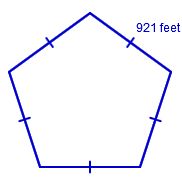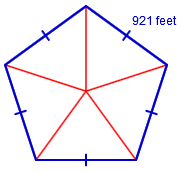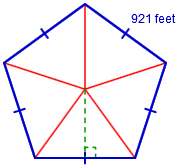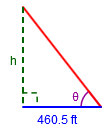### Home > GC > Chapter 12 > Lesson 12.2.1 > Problem12-57

12-57.

The United States Department of Defense is located in a building called the Pentagon because it is in the shape of a regular pentagon. Known as “the largest office building in the world,” its exterior edges measure $921$ feet. Find the area of land enclosed by the outer walls of the Pentagon building.Find the area using trigonometric ratios.The interior angles of a regular pentagon measure $\frac{180(5-2)}{5}=\frac{180\cdot3}{5}=\frac{540}{5}=\ 108º$

$\theta\ =\frac{108º}{2}$